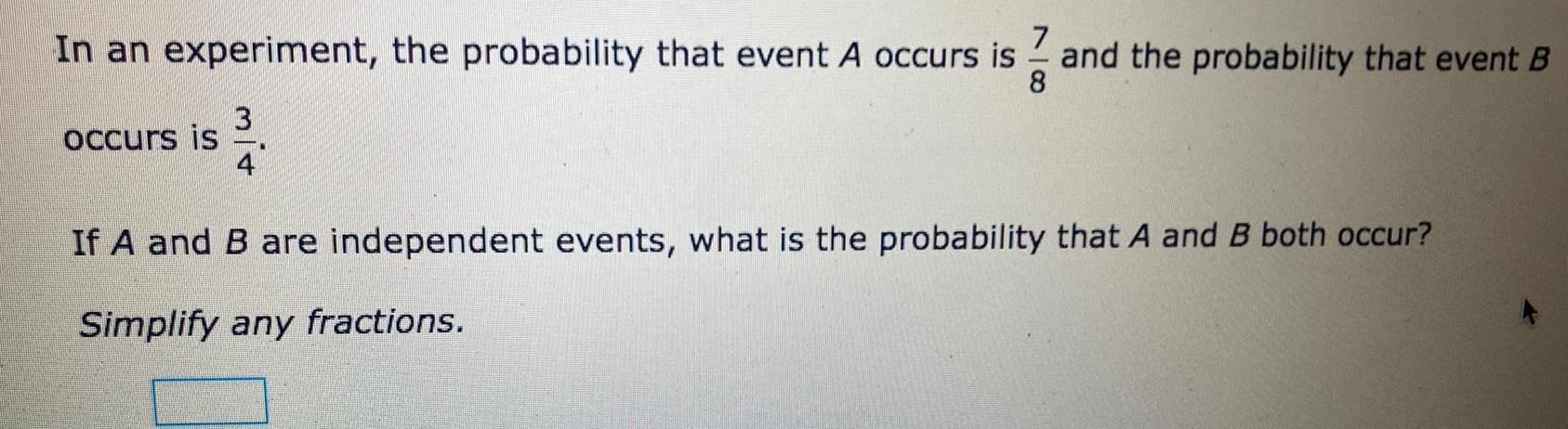Question:

# 7 In an experiment the probability that event A occurs is

Last updated: 9/11/20237 In an experiment the probability that event A occurs is and the probability that event B 8 occurs is 334 If A and B are independent events what is the probability that A and B both occur Simplify any fractions